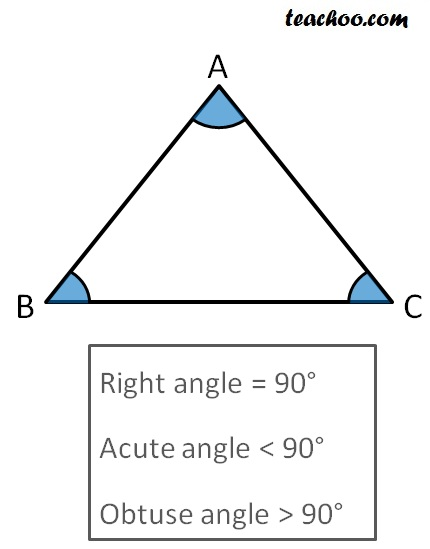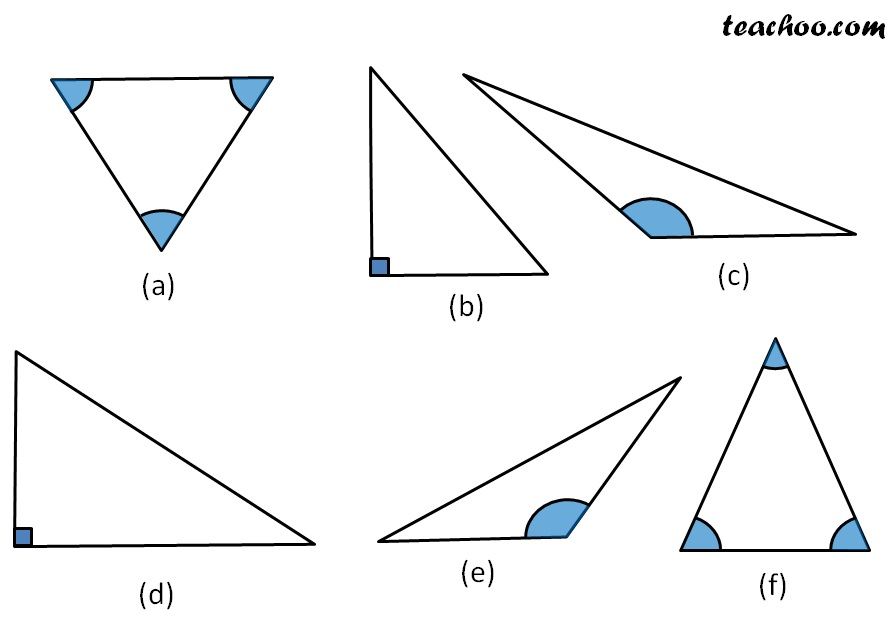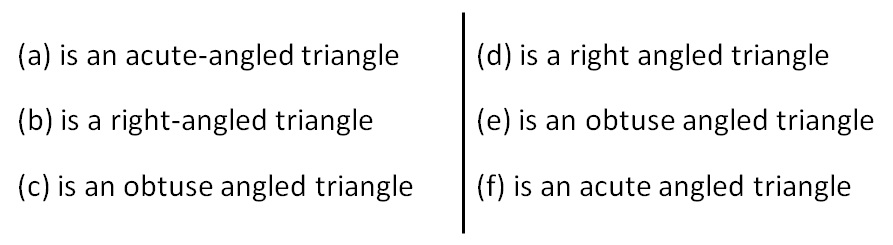Classifying triangles

Chapter 5 Class 6 Understanding Elementary Shapes
Concept wise

##googletag.cmd.push(function() { googletag.display('div-gpt-ad-1669298377854-0'); });

Now, in ∆ABC,

there can be 3 cases

• All angles are acute
• One angle is right angle (90°)
• One angle is greater than 90° (i.e one angle is obtuse)

If all angles are acute, it is an acute angled triangle.

If one angle is right angle, it is a right angled triangle.

If one angle is greater than 90°, it is an obtuse angled triangle.Learn in your speed, with individual attention - Teachoo Maths 1-on-1 Class

### Transcript

Now, in ∆ABC, there can be 3 cases All angles are acute One angle is right angle (90°) One angle is greater than 90° (i.e one angle is obtuse) If all angles are acute, it is an acute angled triangle. If one angle is right angle, it is a right angled triangle. If one angle is greater than 90°, it is an obtuse angled triangle. (a) is an acute-angled triangle (b) is a right-angled triangle (c) is an obtuse angled triangle (d) is a right angled triangle (e) is an obtuse angled triangle (f) is an acute angled triangle